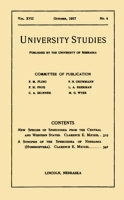## University Studies of the University of Nebraska## Papers from the University Studies series (The University of Nebraska)

#### Date of this Version

1-1905

1905 by The University of Nebraska Printed in the United States by the University 01 Nebraska Printing Division

#### Abstract

When light polarized at an angle of forty-five degrees to the; lines of force is passed through certain dielectrics, between two parallel electrodes, they become double-refracting. The difference of phase, 0, between the two components of light at right angles and parallel to the lines of force, as represented by Kerr and verified by later investigators, is

8=+_BP2l

a2

where B is the electro-optic constant, depending on the dielectric, l the length, and a the distance between the plate electrodes in centimeters, and P the difference of potential between the electrodes in e.G.S. units.

From data obtained in this investigation, there seems to be a decided variation from the above law for low potentials. As the potential was decreased from about 200 volts per millimeter of distance between the electrodes, the decrease of 0 was in a smaller ratio than the decrease of P2.

COinS